Problem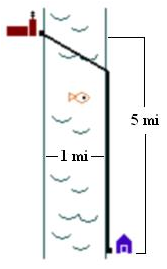You want to run an underground power cable from a power station on one side of a river to a house on the other side, as in the diagram.

The house is 5 miles downstream from the station, and the river has a constant width of 1 mile. It costs \$1000 per mile to lay cable underground, and \$3000 per mile to lay cable under water.

How should you lay the cable to minimize the total cost, and what will the minimum cost be?

Solution

Let x be the amount of cable that is run underground, and y be the amount run under the river. These variables satisfy the equation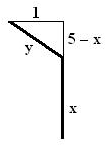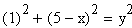and we can write y in terms of x.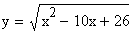Therefore, we can write the cost C as a function of x.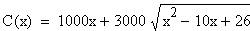It is this function, the total cost of the cable, that we want to minimize. To do this, take the derivative of C(x), set it equal to zero, and solve for x.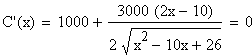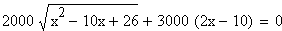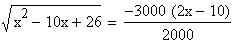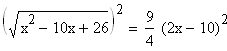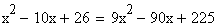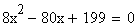Using the quadratic formula or a calculator, we find the solutions are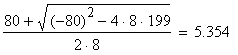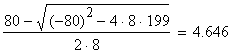The corresponding values for y are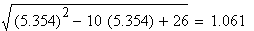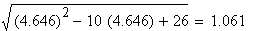Since the distance under the river is the same for both values of x, we choose the smaller x to get a lower cost.

So, the minimum cost will be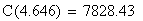This is achieved by laying approximately 4.646 miles of cable upstream from the house along the side of the river, then approximately 1.061 miles underwater to the power plant.